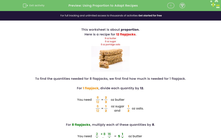# Using Proportion to Adapt Recipes

In this worksheet, students must use proportion to adapt recipes.Key stage:  KS 2

Curriculum topic:   Ratio and Proportion

Curriculum subtopic:   Solve Ratio Problems

Difficulty level:#### Worksheet Overview

Here is a recipe for 12 flapjacks.

• 8 oz butter
• 6 oz sugar
• 6 oz porridge oatsTo find the quantities needed for 8 flapjacks, we first find how much is needed for 1 flapjack.

For 1 flapjack, divide each quantity by 12.

You need
 8 12
=
 2 3
oz butter

 6 12
=
 1 2
oz sugar and
 1 2
oz oats.

For 8 flapjacks, multiply each of these quantities by 8.

You need
 2 3
× 8  =
 16 3
=  5
 1 3
oz butter

 1 2
× 8  =  4 oz sugar and 4 oz oats.

Alternatively, work out 8 ÷ 12 = 2/3, so find 2/3 of each quantity.

### What is EdPlace?

We're your National Curriculum aligned online education content provider helping each child succeed in English, maths and science from year 1 to GCSE. With an EdPlace account you’ll be able to track and measure progress, helping each child achieve their best. We build confidence and attainment by personalising each child’s learning at a level that suits them.

Get started